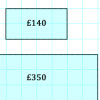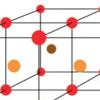# Resources tagged with: Creating and manipulating expressions and formulae

Filter by: Content type:
Age range:
Challenge level:

### There are 58 results

Broad Topics > Algebraic expressions, equations and formulae > Creating and manipulating expressions and formulae### The Number Jumbler

##### Age 7 to 14Challenge Level

The Number Jumbler can always work out your chosen symbol. Can you work out how?### Reversals

##### Age 11 to 14Challenge Level

Where should you start, if you want to finish back where you started?### Think of Two Numbers

##### Age 11 to 14Challenge Level

Think of two whole numbers under 10, and follow the steps. I can work out both your numbers very quickly. How?### More Mathematical Mysteries

##### Age 11 to 14Challenge Level

Write down a three-digit number Change the order of the digits to get a different number Find the difference between the two three digit numbers Follow the rest of the instructions then try. . . .### Magic Squares for Special Occasions

##### Age 11 to 16

This article explains how to make your own magic square to mark a special occasion with the special date of your choice on the top line.### Seven Up

##### Age 11 to 14Challenge Level

The number 27 is special because it is three times the sum of its digits 27 = 3 (2 + 7). Find some two digit numbers that are SEVEN times the sum of their digits (seven-up numbers)?### Quick Times

##### Age 11 to 14Challenge Level

32 x 38 = 30 x 40 + 2 x 8; 34 x 36 = 30 x 40 + 4 x 6; 56 x 54 = 50 x 60 + 6 x 4; 73 x 77 = 70 x 80 + 3 x 7 Verify and generalise if possible.### Chocolate Maths

##### Age 11 to 14Challenge Level

Pick the number of times a week that you eat chocolate. This number must be more than one but less than ten. Multiply this number by 2. Add 5 (for Sunday). Multiply by 50... Can you explain why it. . . .### Number Pyramids

##### Age 11 to 14Challenge Level

Try entering different sets of numbers in the number pyramids. How does the total at the top change?##### Age 11 to 14Challenge Level

If you take a three by three square on a 1-10 addition square and multiply the diagonally opposite numbers together, what is the difference between these products. Why?### Is it Magic or Is it Maths?

##### Age 11 to 14Challenge Level

Here are three 'tricks' to amaze your friends. But the really clever trick is explaining to them why these 'tricks' are maths not magic. Like all good magicians, you should practice by trying. . . .##### Age 11 to 14Challenge Level

Visitors to Earth from the distant planet of Zub-Zorna were amazed when they found out that when the digits in this multiplication were reversed, the answer was the same! Find a way to explain. . . .### Special Numbers

##### Age 11 to 14Challenge Level

My two digit number is special because adding the sum of its digits to the product of its digits gives me my original number. What could my number be?### Why 8?

##### Age 11 to 14Challenge Level

Choose any four consecutive even numbers. Multiply the two middle numbers together. Multiply the first and last numbers. Now subtract your second answer from the first. Try it with your own. . . .### How Many Miles to Go?

##### Age 11 to 14Challenge Level

How many more miles must the car travel before the numbers on the milometer and the trip meter contain the same digits in the same order?### Always a Multiple?

##### Age 11 to 14Challenge Level

Think of a two digit number, reverse the digits, and add the numbers together. Something special happens...### Crossed Ends

##### Age 11 to 14Challenge Level

Crosses can be drawn on number grids of various sizes. What do you notice when you add opposite ends?### Special Sums and Products

##### Age 11 to 14Challenge Level

Find some examples of pairs of numbers such that their sum is a factor of their product. eg. 4 + 12 = 16 and 4 × 12 = 48 and 16 is a factor of 48.##### Age 7 to 14Challenge Level

Think of a number and follow the machine's instructions... I know what your number is! Can you explain how I know?### The Simple Life

##### Age 11 to 14Challenge Level

The answer is $5x+8y$... What was the question?##### Age 11 to 14Challenge Level

Think of a number and follow my instructions. Tell me your answer, and I'll tell you what you started with! Can you explain how I know?### Diagonal Sums

##### Age 7 to 14Challenge Level

In this 100 square, look at the green square which contains the numbers 2, 3, 12 and 13. What is the sum of the numbers that are diagonally opposite each other? What do you notice?### Top-heavy Pyramids

##### Age 11 to 14Challenge Level

Use the numbers in the box below to make the base of a top-heavy pyramid whose top number is 200.### Magic Sums and Products

##### Age 11 to 16

How to build your own magic squares.### Algebra Match

##### Age 11 to 16Challenge Level

A task which depends on members of the group noticing the needs of others and responding.### Fibonacci Surprises

##### Age 11 to 14Challenge Level

Play around with the Fibonacci sequence and discover some surprising results!##### Age 11 to 14Challenge Level

Think of a number, add one, double it, take away 3, add the number you first thought of, add 7, divide by 3 and take away the number you first thought of. You should now be left with 2. How do I. . . .### Price Match

##### Age 7 to 11Challenge Level

Can you find pairs of differently sized windows that cost the same?##### Age 11 to 14Challenge Level

Surprising numerical patterns can be explained using algebra and diagrams...### Always the Same

##### Age 11 to 14Challenge Level

Arrange the numbers 1 to 16 into a 4 by 4 array. Choose a number. Cross out the numbers on the same row and column. Repeat this process. Add up you four numbers. Why do they always add up to 34?### Algebra from Geometry

##### Age 11 to 16Challenge Level

Account of an investigation which starts from the area of an annulus and leads to the formula for the difference of two squares.### Good Work If You Can Get It

##### Age 11 to 14Challenge Level

A job needs three men but in fact six people do it. When it is finished they are all paid the same. How much was paid in total, and much does each man get if the money is shared as Fred suggests?### Legs Eleven

##### Age 11 to 14Challenge Level

Take any four digit number. Move the first digit to the end and move the rest along. Now add your two numbers. Did you get a multiple of 11?##### Age 11 to 14Challenge Level

A little bit of algebra explains this 'magic'. Ask a friend to pick 3 consecutive numbers and to tell you a multiple of 3. Then ask them to add the four numbers and multiply by 67, and to tell you. . . .### Sum Equals Product

##### Age 11 to 14Challenge Level

The sum of the numbers 4 and 1 [1/3] is the same as the product of 4 and 1 [1/3]; that is to say 4 + 1 [1/3] = 4 ï¿½ 1 [1/3]. What other numbers have the sum equal to the product and can this be. . . .##### Age 11 to 14Challenge Level

List any 3 numbers. It is always possible to find a subset of adjacent numbers that add up to a multiple of 3. Can you explain why and prove it?### Even So

##### Age 11 to 14Challenge Level

Find some triples of whole numbers a, b and c such that a^2 + b^2 + c^2 is a multiple of 4. Is it necessarily the case that a, b and c must all be even? If so, can you explain why?### Sums of Pairs

##### Age 11 to 16Challenge Level

Jo has three numbers which she adds together in pairs. When she does this she has three different totals: 11, 17 and 22 What are the three numbers Jo had to start with?”### Hot Pursuit

##### Age 11 to 14Challenge Level

I added together the first 'n' positive integers and found that my answer was a 3 digit number in which all the digits were the same...### Card Trick 1

##### Age 11 to 14Challenge Level

Can you explain how this card trick works?### Regular Hexagon Loops

##### Age 11 to 14Challenge Level

Make some loops out of regular hexagons. What rules can you discover?### Summing Consecutive Numbers

##### Age 11 to 14Challenge Level

15 = 7 + 8 and 10 = 1 + 2 + 3 + 4. Can you say which numbers can be expressed as the sum of two or more consecutive integers?### Partitioning Revisited

##### Age 11 to 14Challenge Level

We can show that (x + 1)² = x² + 2x + 1 by considering the area of an (x + 1) by (x + 1) square. Show in a similar way that (x + 2)² = x² + 4x + 4### How Much Can We Spend?

##### Age 11 to 14Challenge Level

A country has decided to have just two different coins, 3z and 5z coins. Which totals can be made? Is there a largest total that cannot be made? How do you know?### Hallway Borders

##### Age 11 to 14Challenge Level

What are the possible dimensions of a rectangular hallway if the number of tiles around the perimeter is exactly half the total number of tiles?### Boxed In

##### Age 11 to 14Challenge Level

A box has faces with areas 3, 12 and 25 square centimetres. What is the volume of the box?### Perimeter Expressions

##### Age 11 to 14Challenge Level

Create some shapes by combining two or more rectangles. What can you say about the areas and perimeters of the shapes you can make?### Beach Huts

##### Age 11 to 14Challenge Level

Can you figure out how sequences of beach huts are generated?### Finding 3D Stacks

##### Age 7 to 11Challenge Level

Can you find a way of counting the spheres in these arrangements?### Can They Be Equal?

##### Age 11 to 14Challenge Level

Can you find rectangles where the value of the area is the same as the value of the perimeter?